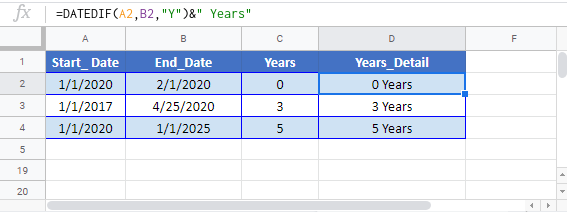# Number of Years Between Dates in Excel & Google Sheets

This tutorial will demonstrate how to calculate the number of years between two dates in Excel & Google Sheets.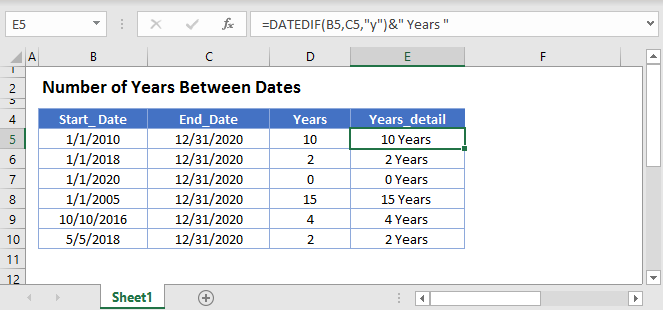## DATEDIF Function

To calculate the number of years between dates you can use the DATEDIF Function with unit of measurement “y” for years: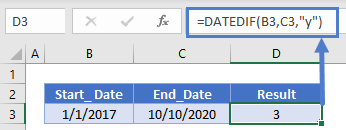The DATEDIF Function returns the number of full years between two dates. It will not give you a fractional value.

Next you can use the “ym” input with the DATEDIF function to return the remaining number of months: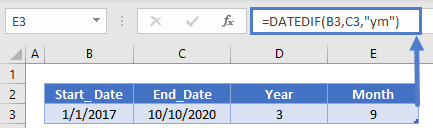and join them together to output the number of years and months between two dates: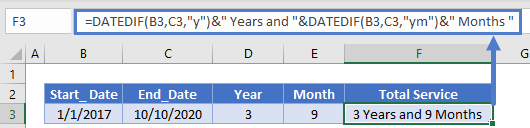## YEARFRAC Function

Instead if you want to determine the number of years, including fractional values you can use the YEARFRAC Function: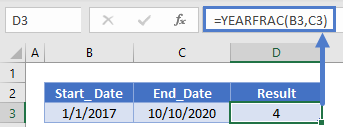You can add in the TRUNC or INT Functions to calculate the number of full years (just like the DATEDIF Function):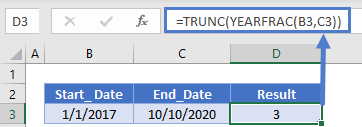Or you can use the ROUND Function to round to the nearest year: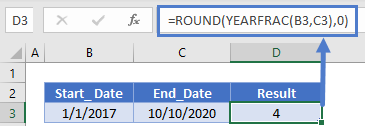Last, you can use the ROUNDUP Function to count any partial year as a full year: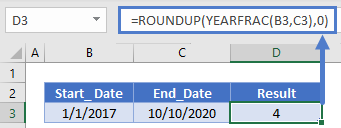Number of Years Between Dates in Google Sheets
All of the above examples work exactly the same in Google Sheets as in Excel.